2021-10-01 19:28

# c语言冒泡排序相关问题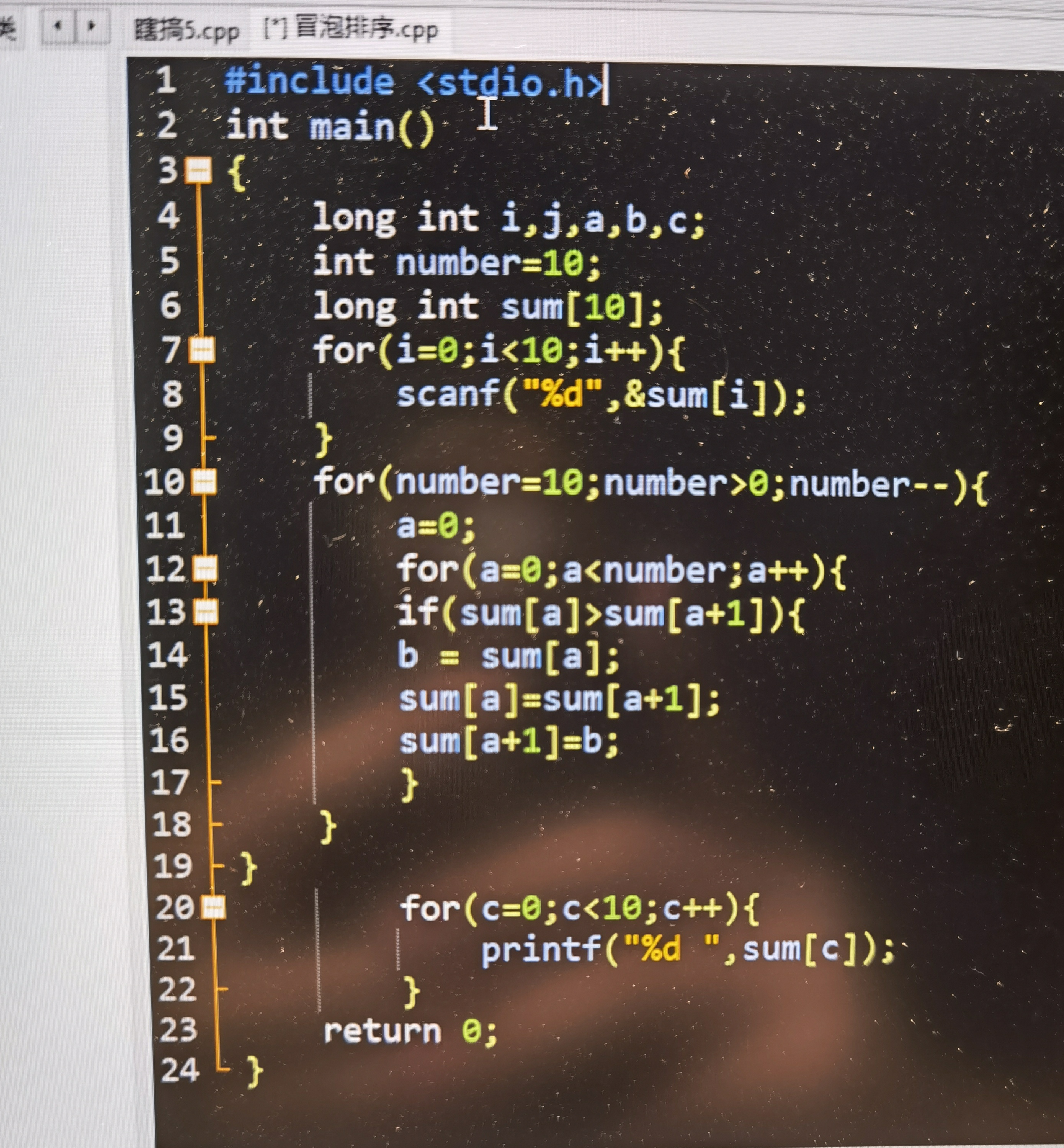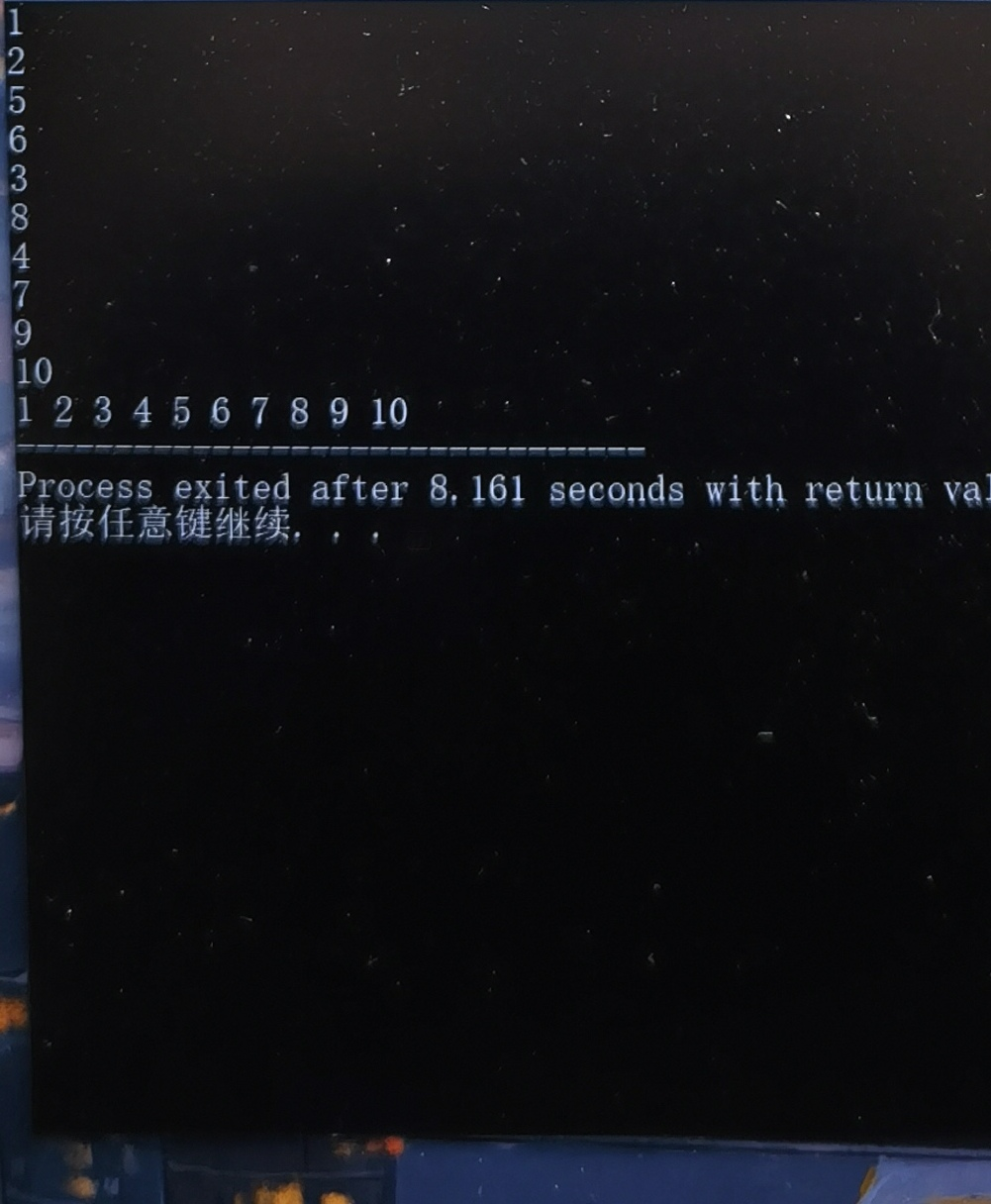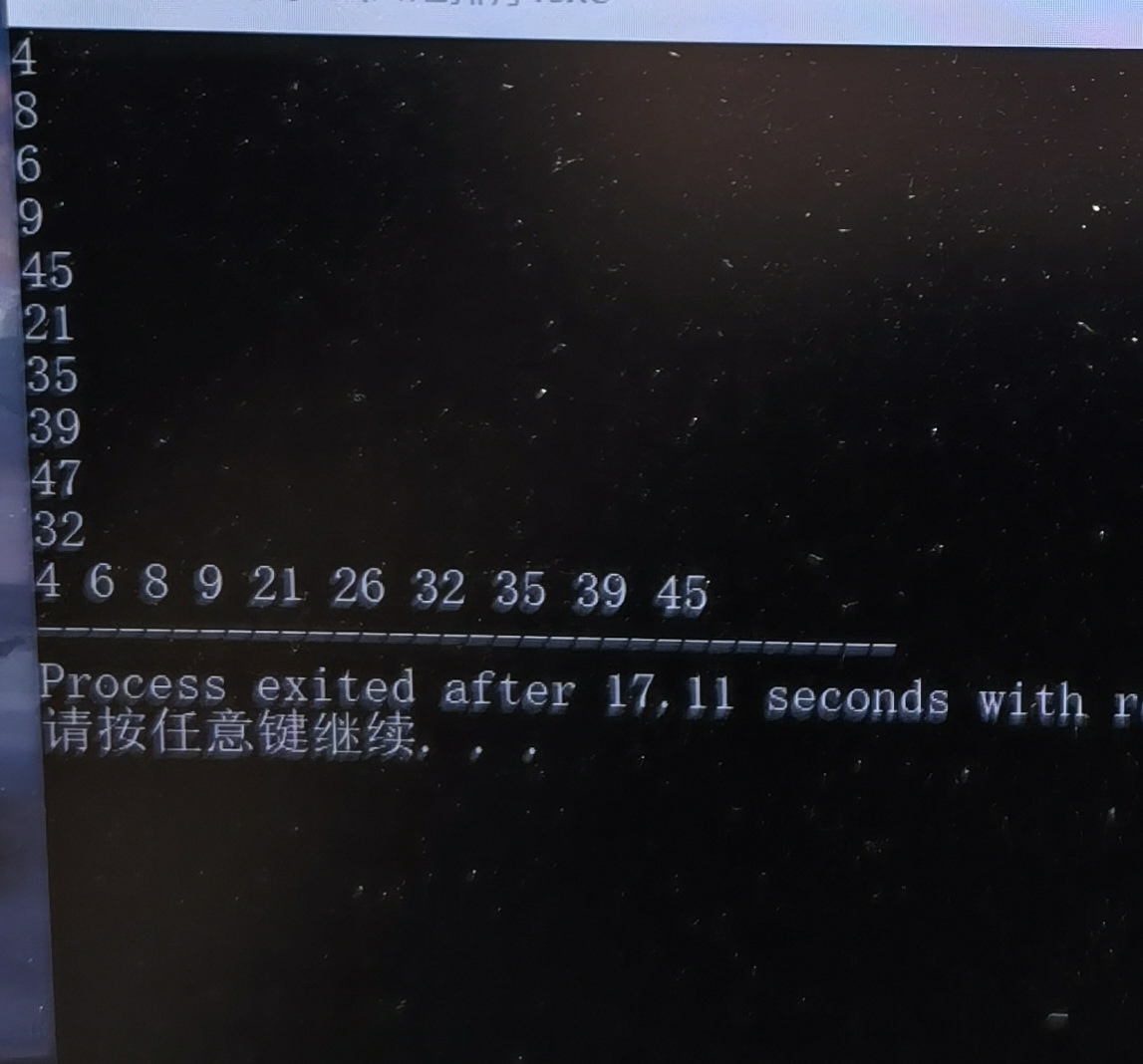#### 图片转代码服务由CSDN问答提供 功能建议

`````` 10
1
2345678910
Process exited after8.161secondswithreturnva
请按任意键继续``````
• 好问题 提建议
• 收藏

#### 2条回答默认 最新

•19ty53 2021-10-01 19:33
已采纳

既然你开的数组是10，那么你应该使用0-9的下标。不过当溢出量不多时C++会自动申请后面的很少的连续地址。
不过我想告诉你的是，如果你在主函数里面开数组。那就是从栈中开空间，但是栈中是会有上一次别的进程的残余，所以如果你没有输入，就一定要初始化，不然就会出现一些你不知道的东西（别的进程的垃圾），你用的是地址1-10。0地址你没有用，然而你只是给1~10的地址输入赋了初值，0地址没有，所以就会出现上一次的垃圾，这个遗留垃圾的ASCLL码是26

已采纳该答案
评论
解决 无用
打赏 举报
•19ty53 2021-10-01 20:02
``````
#include<stdio.h>
int main(){
long int i,j,a,b,c;
int number=10;
long int sum;
for(i=0;i<10;i++){
scanf("%d",&sum[i]);
}
for(number=10;number>0;number--){
a=0;
for(a=0;a<number;a++){
if(sum[a]>sum[a+1]){
b=sum[a];
sum[a]=sum[a+1];
sum[a+1]=b;
}
}
}
for(c=0;c<10;c++){
printf("%d ",sum[c]);
}
return 0;
}
``````

如果我用你的代码。
就会出现垃圾内存34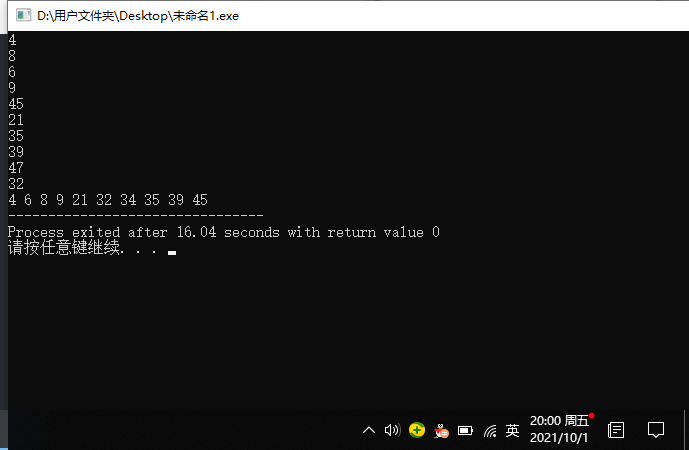如果我改成这个

``````#include<stdio.h>
int main(){
long int i,j,a,b,c;
int number=10;
long int sum;
for(i=0;i<10;i++){
scanf("%d",&sum[i]);
}
for(number=9/*这里*/;number>0;number--){
a=0;
for(a=0;a<number;a++){
if(sum[a]>sum[a+1]){
b=sum[a];
sum[a]=sum[a+1];
sum[a+1]=b;
}
}
}
for(c=0;c<10;c++){
printf("%d ",sum[c]);
}
return 0;
}
``````

那就是正确答案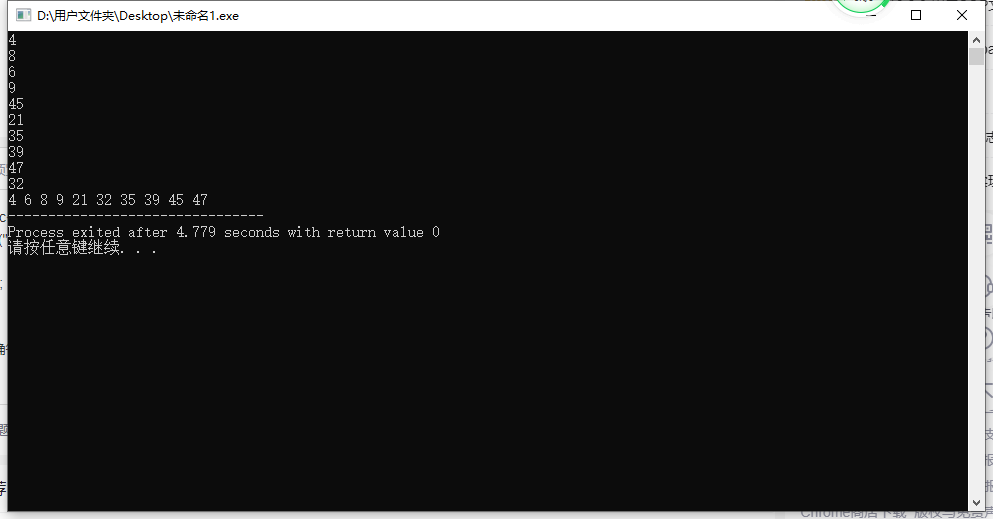评论
解决 无用
打赏 举报